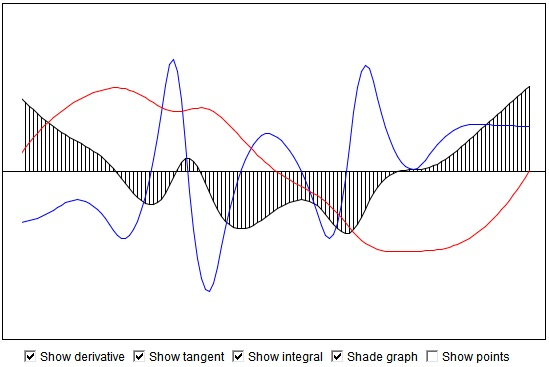Function, Derivative and Integral

Points on the graph below are draggable up and down. So that you can modify the function any other way you want. Simultaneously with the function, you may display its derivative and the integral as a function of its upper limit. See if you can relate the behavior of the function to the behaviors of its derivative and the integral. If you drag the cursor while the "Show tangent" box is checked a short line will be displayed tangent to either graph of the function or the graph of the integral.

As of 2018, Java plugins are not supported by any browsers (find out more). This Wolfram Demonstration, Polynomial and Derivative, shows an item of the same or similar topic, but is different from the original Java applet, named 'SplineTest'. The originally given instructions may no longer correspond precisely.

(image below from deprecated 'SplineTest' applet)The graph is a natural cubic spline passing at the outset through 11 points. The points are added as needed whenever you attempt to drag the graph in-between the existing points.• What Is Line?
• Functions, what are they?
• Cartesian Coordinate System
• Addition and Subtraction of Functions
• Graph of a Polynomial of arbitrary degree
• Graph of a Polynomial Defined by Its Roots
• Inflection Points of Fourth Degree Polynomials
• Lagrange Interpolation (an Interactive Gizmo)
• Equations of a Straight Line
• Taylor Series Approximation to Cosine
• Taylor Series Approximation to Cosine
• Linear Function with Coefficients in Arithmetic Progression
• Sine And Cosine Are Continuous Functions
•